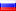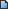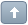## Live Production Software Forums

Welcome Guest! To enable all features please Login or Register.Error

 Previous Topic Next Topic
 svetotehnik #1 Posted : Friday, June 17, 2022 2:09:29 AM(UTC)Rank: MemberGroups: Registered Joined: 11/6/2020(UTC)Posts: 20Location: Saint-PetersburgThanks: 5 times There is a game, written on vb.netYou can use source code or just download .rar, import configuration and try itRecommended font for score is Connection IIgame v1.rar (34kb) downloaded 20 time(s).Code:``````dim xml_for_best as string = API.XML() dim x_for_best as new system.xml.xmldocument x_for_best.loadxml(xml_for_best) dim last_best double = 0 dim Xbar as string = "0" dim barXd as double = 0 dim WhatISX as double = 0 dim WhatISY as double = 0 dim angle as double = 1 dim dirX as int32 = 1 dim dirY as int32 = 1 dim score as double = 0 dim delay as double = 20 'check if there is a better score, if not, write 0 If Not ((x_for_best.SelectSingleNode("//dynamic/value2").InnerText) Is Nothing) Then dim best as int32 = 0 Else last_best = (x_for_best.SelectSingleNode("//dynamic/value2").InnerText) End If 'put the racket on stop API.Function("SetDynamicValue1", Value:="S") 'write 0 for score API.Function("SetText", Input:="score.gtzip", SelectedIndex:="0", Value:=score) While True dim xml as string = API.XML() dim x as new system.xml.xmldocument x.loadxml(xml) 'check the commands for the movement of the racket dim LRbar as string = (x.SelectSingleNode("//dynamic/value1").InnerText) If LRbar = "L" Then barXd=barXd-0.01 End If If LRbar = "R" Then barXd=barXd+0.01 End If 'stop the racket if it reaches the edge of the screen If barXd < -0.839 Then API.Function("SetDynamicValue1", Value:="S") End If If barXd > 0.839 Then API.Function("SetDynamicValue1", Value:="S") End If 'converting the data in suitable for API dim barXs as string = barXd.ToString If barXs.Contains(",") Then barXs = barXs.Replace(",", ".") End If 'racket movement API.Function("SetPanX", Input:="player_bar.png", SelectedIndex:="0", Value:=barXs) dim WhatXbarD as double = Xbar WhatISX=WhatISX-0.01*dirX WhatISY=WhatISY+0.01*dirY*angle If WhatISX < -0.954 Then dirX = -1 End If If WhatISY > 1.662 Then dirY = -1 End If If WhatISX > 0.954 Then dirX = 1 End If If WhatISY < -0.001 Then 'game over If barXd-0.176 > WhatISX dim last_best_d as double = Convert.ToDouble(last_best) If score > last_best_d Then API.Function("SetText", Input:="score.gtzip", SelectedIndex:="1", Value:=score) API.Function("SetDynamicValue2", Value:="score") End If API.Function("OverlayInput3In", Input:="game_over.png") API.Function("ScriptStopAll") ElseIf barXd+0.176 < WhatISX dim last_best_d as double = Convert.ToDouble(last_best) If score > last_best_d Then API.Function("SetText", Input:="score.gtzip", SelectedIndex:="1", Value:=score) API.Function("SetDynamicValue2", Value:="score") End If API.Function("OverlayInput3In", Input:="game_over.png") API.Function("ScriptStopAll") Else 'score increment score = score+100 API.Function("SetText", Input:="score.gtzip", SelectedIndex:="0", Value:=score) If delay > 5 Then If LRbar = "L" and dirX = 1 Then angle = angle*1.2 ElseIf LRbar = "L" and dirX = -1 Then angle = angle/1.3 ElseIf LRbar = "R" and dirX = 1 Then angle = angle*1.4 ElseIf LRbar = "R" and dirX = -1 Then angle = angle/1.1 End If dirY = 1 'speed increase delay = delay - 1 Else If LRbar = "L" and dirX = 1 Then angle = angle*1.2 ElseIf LRbar = "L" and dirX = -1 Then angle = angle/1.3 ElseIf LRbar = "R" and dirX = 1 Then angle = angle*1.4 ElseIf LRbar = "R" and dirX = -1 Then angle = angle/1.1 End If dirY = 1 'speed decrease delay = delay + 10 End If End If End If 'converting the data in suitable for API dim WhatISXs as string = WhatISX.ToString dim WhatISYs as string = WhatISY.ToString If WhatISXs.Contains(",") Then WhatISXs = WhatISXs.Replace(",", ".") End If If WhatISYs.Contains(",") Then WhatISYs = WhatISYs.Replace(",", ".") End If 'ball movement API.Function("SetPanX", Input:="ball.png", SelectedIndex:="0", Value:=WhatISXs) API.Function("SetPanY", Input:="ball.png", SelectedIndex:="0", Value:=WhatISYs) sleep(delay) End While``````Wanna join the discussion?! Login to your vMix Forums forum account, or Register a new forum account.Users browsing this topic
Forum Jump
You cannot post new topics in this forum.
You cannot reply to topics in this forum.
You cannot delete your posts in this forum.
You cannot edit your posts in this forum.
You cannot create polls in this forum.
You cannot vote in polls in this forum.